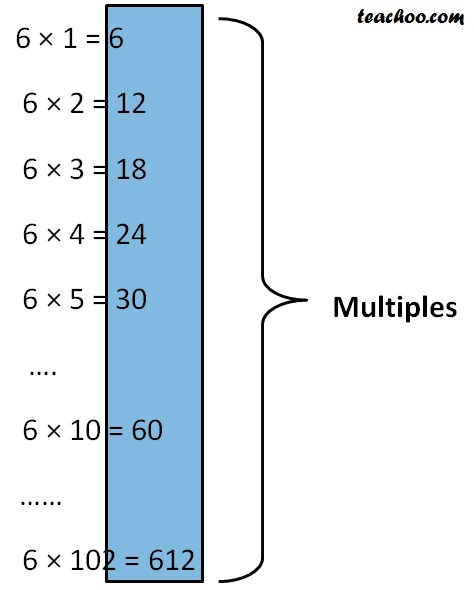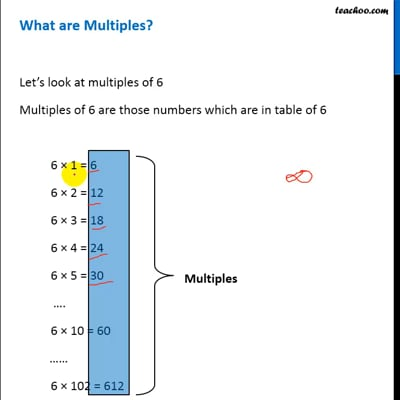Factors and Multiples

Chapter 3 Class 6 Playing with Numbers
Concept wise

Let’s look at multiples of 6

Multiples of 6 are those numbers which are in table of 6All these are Multiples

## Properties of multiples

• There can be infinite number of multiples
(Infinite means No limit )
• Smallest Multiple is Number itself (6)
• Multiples are greater than or equal to number
(6, 12, 18, 24, … are all greater than or equal to 6)This video is only available for Teachoo black users

### Transcript

6 × 1 = 6 6 × 2 = 12 6 × 3 = 18 6 × 4 = 24 6 × 5 = 30 …. 6 × 10 = 60 …… 6 × 102 = 612